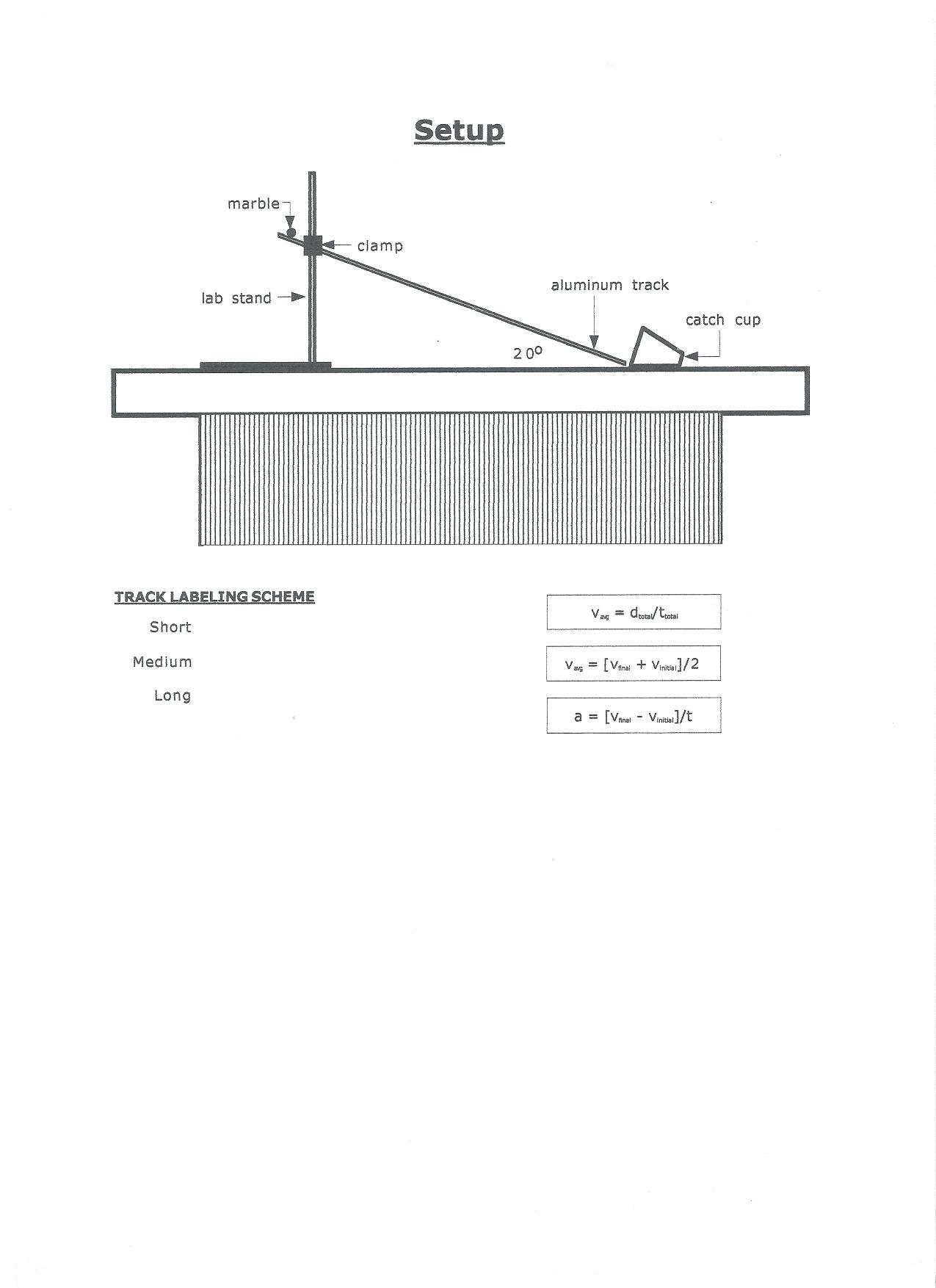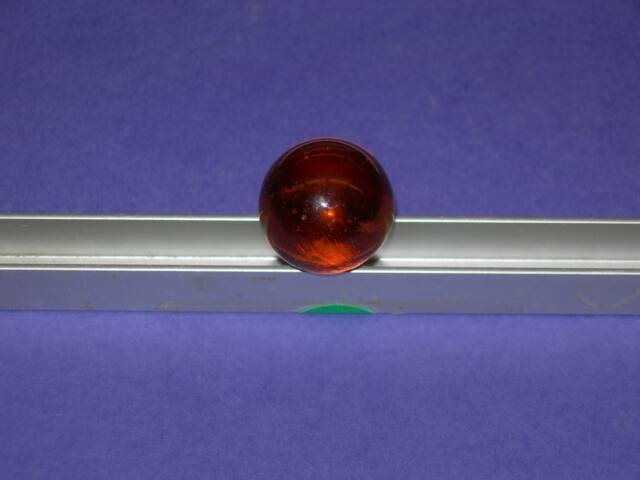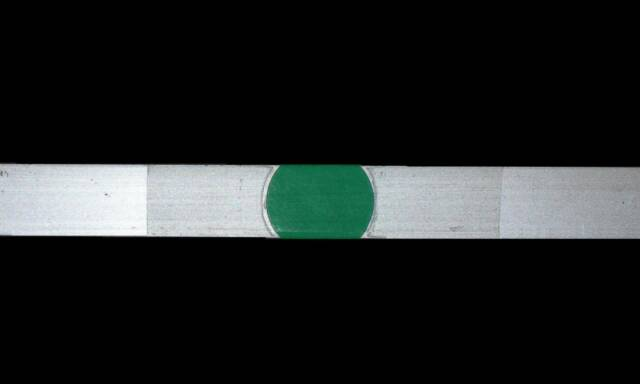Cycle I Regents Physics
John Dewey High School
Mr. Klimetz
Uniform Linear Accelerated Motion
Laboratory No. 6
Introduction. The motion of a smooth spherical object descending an inclined linear trackway can be considered to be experiencing uniform linear acceleration under ordinary laboratory circumstances, that is, if frictional effects are neglected. The purpose of this activity is to acquaint you with the kinematic features of uniform linear accelerated motion as exemplified by the travel of a polished glass sphere down three linear trackways of differing length inclined at the same angle with respect to the horizontal. Through the use of the equations for determining average speed, it is possible to determine the final speed and hence the acceleration of the sphere.
Procedure, Data and Data Analysis.1. Measure and record the following features:
Equipment.Short-length aluminum C-channel linear trackwayMedium-length aluminum C-channel linear trackwayLong-length aluminum C-channel linear trackway2.5-cm-diameter polished glass sphere8 fluid ounce styrofoam cup half-filled with cotton or fiberglass waddingMeter stickStopwatchProtractorGraph paperCalculatorLab stand and c-clamp
Objectives. The objectives of this laboratory exercise are to acquaint you with the physical behavior of an object experiencing uniform linear accelerated motion and to promote a clear conceptual and mathematical understanding of the kinematics of such motion. Through the application of basic Newtonian kinematic principles governing ULAM to the measurement of the distance, travel time and initial speed of an object descending three inclined linear trackways of differing length, you are expected to determine the object'saverage speed (vavg),final speed (vf), andacceleration (a).

An Exercise in the Versatility and Usefulness of the Equations of Average Speed
Short-Length Trackway
Distance __________ m (dM)
Distance __________ m (dS)
Distance __________ m (dL)
Medium-Length Trackway
Long-Length Trackway2.  Elevate one end of each trackway by placing it on the c-clamp attached to the upright of the lab stand while the other end rests on the lab table. Place a 3-cm-thick layer of cotton (or fiberglass wadding) at the bottom of the styrofoam cup. Turn the cup sideways and slide the lower end of the trackway into the cup so that the end of the trackway just contacts the wadding. Using your protractor, adjust your trackway so that it is inclined 10 degrees with respect to the horizontal lab table top. Place the glass sphere at the uppermost end of the trackway and hold. Release the glass sphere and, with the stopwatch provided, measure the amount of time it takes for it to travel down the trackway and into the cup. This should be done thrice (three times) for each trackway. This is the travel time of the sphere. Record your travel times on the lines below to the 1/100th of a second.
Short-Length Trackway:
Time Trial 1 __________ s (t1)
Time Trial 2 __________ s (t2)
Time Trial 3 __________ s (t3)
Medium-Length Trackway:
Time Trial 1 __________ s (t1)
Time Trial 2 __________ s (t2)
Time Trial 3 __________ s (t3)
Long-Length Trackway
Time Trial 1 __________ s (t1)
Time Trial 2 __________ s (t2)
Time Trial 3 __________ s (t3)3.  Calculate the average travel time for each trackway and record on the lines below. Show all work.
Calculations:
Short-Length Trackway:
Average Time __________ s (tavgS)
Medium-Length Trackway:
Average Time __________ s (tavgM)
Long-Length Trackway
Average Time __________ s t(avgL)4.  Calculate the average speed of the sphere for each trackway and record on the lines below. Show all work.
Short-Length Trackway:
Average Speed __________ m/s (vavg)
Calculations:
tavgS = (t1 + t2 + t3)/3
vavgS = dS/tavgS
Medium-Length Trackway:
Average Speed __________ m/s (vavg)
Long-Length Trackway
Average Speed __________ m/s (vavg)
vavgM = dM/tavgM
vavgL = dL/tavgL5.  Calculate the final speed (vf) of the sphere for each trackway and record on the lines below. Show all work.
Short-Length Trackway:
Final Speed __________ m/s (vfS)
Calculations:
vavgS = (vfS + vi)/2
Medium-Length Trackway:
Final Speed __________ m/s (vfM)
Long-Length Trackway
Final Speed __________ m/s (vfL)
vavgM = (vfM + vi)/2
vavgL = (vfL + vi)/26.  Calculate the acceleration (a) of the sphere for each trackway and record on the lines below. Show all work.
Short-Length Trackway:
Acceleration __________ m/sexp2 (aS)
Medium-Length Trackway:
Acceleration __________ m/sexp2 (aM)
Long-Length Trackway
Acceleration __________ m/sexp2 (aL)
Calculations:
aS = (vfS - vi)/tavgS
aM = (vfM - vi)/tavgM
aL = (vfL - vi)/tavgL
tavgM = (t1 + t2 + t3)/3
tavgM = (t1 + t2 + t3)/3
Questions

1. Which features of the apparatus (or factors) directly affected the acceleration and which had no apparent
effect? Explain.

2. Briefly express any possible sources of error in this exercise.
3. How do the values of aS, aM, and aL compare? Briefly account for your results and any differences.
4. Plot a v-t graph for each of the trackways. Briefly account for your results and and sources of error.Marble (Glass Sphere Roller) on Short Aluminum TrackUnderside of Short Aluminum Track10